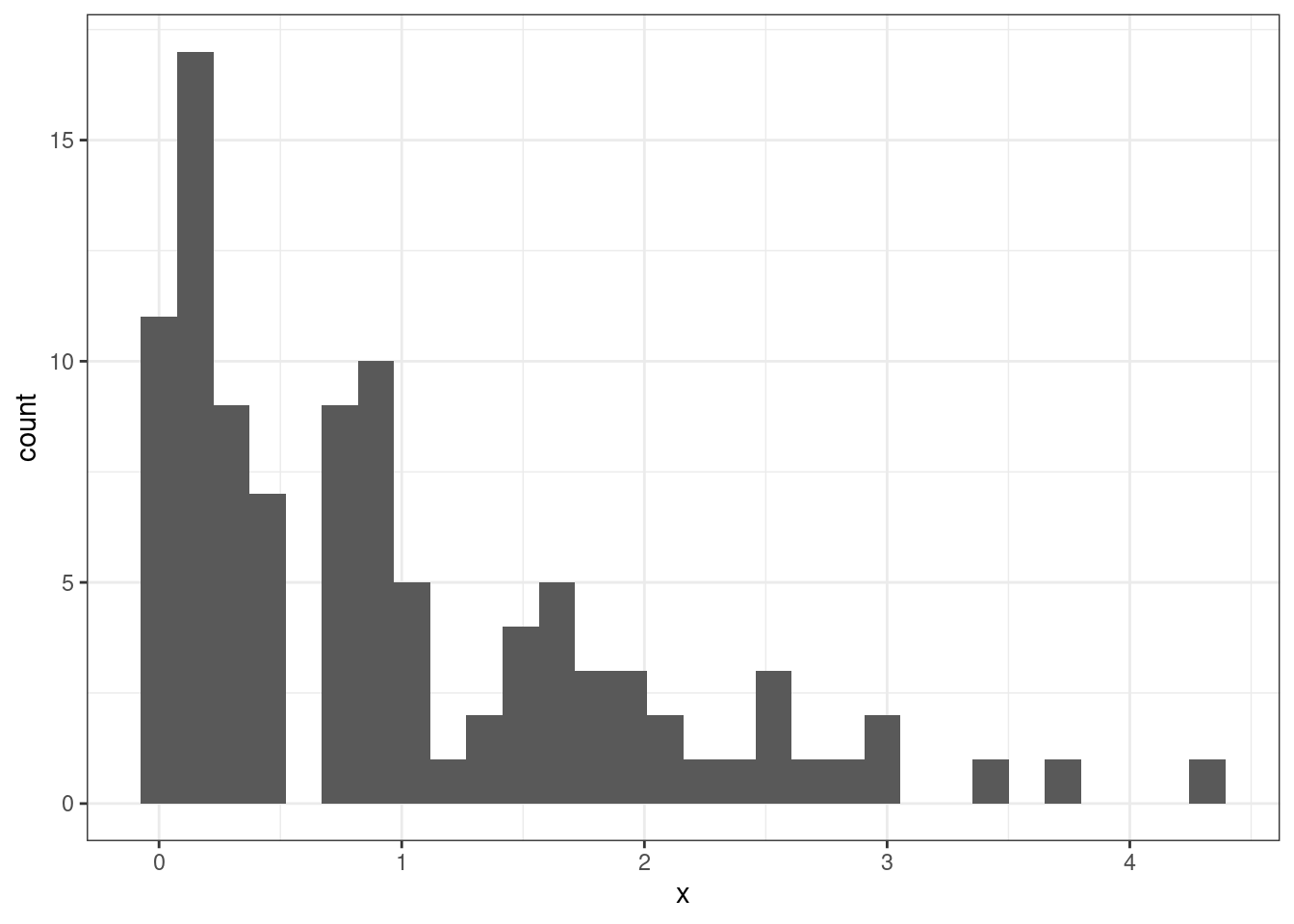# Ch. 3, Coding Question 1

library(ggplot2)
set.seed(1234) # setting the seed means that we will get the same results
x <- rexp(100) # make 100 draws from an exponential distribution

ggplot(data=data.frame(x=x),
mapping=aes(x=x)) +
geom_histogram() +
theme_bw()# Ch. 3, Extra Question 1

Part a

\begin{aligned} \mathbb{E}[Y] &= \mathbb{E}[5+9X] \\ &= \mathbb{E} + \mathbb{E}[9X] \\ &= 5 + 9\mathbb{E}[X] \\ &= 95 \end{aligned}

where the first equality holds by the definition of $$Y$$, the second equality holds because expectations can pass through sums, the third equality holds because the expectation of a constant is just the constant itself and because constants can come outside of expectations, and the last equality holds because $$\mathbb{E}[X]=10$$.

Part b

\begin{aligned} \mathrm{var}(Y) &= \mathrm{var}(5+9X) \\ &= \mathrm{var}(9X) \\ &= 81 \mathrm{var}(X) \\ &= 162 \end{aligned}

where the first equality holds by the definition of $$Y$$, the second equality holds because $$5$$ is a constant and doesn’t contribute to the variance, the third equality holds because constants can come out of the variance (after squaring it), and the last equality holds because $$\mathrm{var}(X)=2$$.

# Ch. 3, Extra Question 2

\begin{aligned} \mathrm{var}(bX) &= \mathbb{E}\big[ (bX - \mathbb{E}[bX])^2 \big] \\ &= \mathbb{E}\big[ (bX)^2 - 2bX\mathbb{E}[bX] + \mathbb{E}[bX]^2 \big] \\ &= \mathbb{E}\big[ b^2X^2 - 2bX\mathbb{E}[bX] + (b\mathbb{E}[X])^2 \big] \\ &= \mathbb{E}\big[ b^2X^2 - 2bX\mathbb{E}[bX] + b^2\mathbb{E}[X]^2 \big] \\ &= \mathbb{E}\big[b^2X^2\big] - \mathbb{E}\big[2bX\mathbb{E}[bX]\big] + \mathbb{E}\big[ b^2 \mathbb{E}[X]^2 \big] \\ &= b^2 \mathbb{E}[X^2] - 2b \mathbb{E}[bX] \mathbb{E}[X] + b^2 \mathbb{E}[X^2] \\ &= b^2 \mathbb{E}[X^2] - 2b^2 \mathbb{E}[X]^2 + b^2 \mathbb{E}[X^2] \\ &= b^2 \mathbb{E}[X^2] - b^2 \mathbb{E}[X]^2 \\ &= b^2 \big(\mathbb{E}[X^2] - \mathbb{E}[X]^2 \big) \\ &= b^2 \mathrm{var}(X) \end{aligned}

where the first equality holds by the definition of variance, the second equality squares the term inside the expectation, the third equality simplifies the first term and pull $$b$$ outside of the expectation for the third term, the fourth equality simplifies the third term, the fifth equality passes the expectation through the sum/difference, the sixth equality pulls constants out of each expectation, the seventh equality pulls one more constant out of an expectation for the middle term, the eight equality combines the second and third terms, the ninth equality factors out $$b^2$$, and the last equality holds because we know that $$\mathrm{var}(X) = \mathbb{E}[X^2] - \mathbb{E}[X]^2$$.

# Ch. 3, Extra Question 3

Part a

\begin{aligned} \mathbb{E}[Y] &= \mathbb{E}[Y|X=1] \mathrm{P}(X=1) + \mathbb{E}[Y|X=0]\mathrm{P}(X=0) \\ &= \mathbb{E}[Y|X=1] \mathrm{P}(X=1) + \mathbb{E}[Y|X=0](1-\mathrm{P}(X=1)) \\ &= 5\' 4\" (0.5) + 5\' 9\" (0.5) \\ &= 5\' 6.5\" \end{aligned}

Part b

The answer from part a is related to the law of iterated expectations because the key step in that problem is to relate the overall expectation, $$\mathbb{E}[Y]$$, to the conditional expectations, $$\mathbb{E}[Y|X=0]$$ and $$\mathbb{E}[Y|X=1]$$. The law of iterated expectations says that unconditional expectations are equal to averages of conditional expectations, which is what we use in the first step of the answer for part a.

# Ch. 3, Extra Question 4

Part a

$$f_X(21) = 0.1$$. We know this because the sum of the pdfs across all possible values of $$X$$ must add up to 1.

Part b

\begin{aligned} \mathbb{E}[X] &= \sum_{x \in \mathcal{X}} x f_X(x) \\ &= 2 f_X(2) + 7 f_X(7) + 13 f_X(13) + 21 f_X(21) \\ &= 2 (0.5) + 7 (0.25) + 13 (0.15) + 21 (0.1) \\ &= 6.8 \end{aligned}

Part c

To calculate the variance, I’ll use the expression $$\mathrm{var}(X) = \mathbb{E}[X^2] - \mathbb{E}[X]^2$$. Thus, the main new thing to calculate is $$\mathbb{E}[X^2]$$:

\begin{aligned} \mathbb{E}[X^2] &= \sum_{x \in \mathcal{X}} x^2 f_X(x) \\ &= 2^2 f_X(2) + 7^2 f_X(7) + 13^2 f_X(13) + 21^2 f_X(21) \\ &= 4 (0.5) + 49 (0.25) + 169 (0.15) + 441 (0.1) \\ &= 83.7 \end{aligned}

Since, we already calculated $$\mathbb{E}[X] = 6.8$$ in part a, this implies that $$\mathbb{E}[X]^2 = 46.24$$. Thus, \begin{aligned} \mathrm{var}(X) &= 83.7 - 46.24 \\ &= 37.46 \end{aligned}

Part d

$F_X(1) = 0$

since the smallest possible value of $$X$$ is 2.

\begin{aligned} F_X(7) &= f_X(2) + f_X(7) \\ &= 0.75 \end{aligned}

\begin{aligned} F_X(8) &= f_X(2) + f_X(7) \\ &= 0.75 \end{aligned}

$F_X(25) = 1$ since all possible values that $$X$$ can take are less than 25.